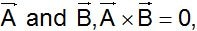# Measurement and Physical Quantities Quiz### MCQs on Physical World and Measurement:

Ques. Two forces of 12 N and 8 N act upon a body. The resultant force on the body has maximum value of
(a) 4 N
(b) 0 N
(c) 20 N
(d) 8 N

Ans. (c)

Ques. A hall has the dimensions 10 m x 12m x 14 m A fly starting at one corner ends up at a diametrically opposite corner. What is the magnitude of its displacement
(a) 17 m
(b) 26 m
(c) 36 m
(d) 20 m

Ans. (d)

Ques. A body moves due East with velocity 20 km/hour and then due North with velocity 15 km/hour. The resultant velocity
(a) 5 km/hour
(b) 15 km/hour
(c) 20 km/hour
(d) 25 km/hour

Ans. (d)

Ques. A man standing on a road hold his umbrella at 30° with the vertical to keep the rain away. He throws the umbrella and starts running at 10 km/hr.

He finds that raindrops are hitting his head vertically, the speed of raindrops with respect to the road will be
(a) 10 km/hr
(b) 20 km/hr
(c) 30 km/hr
(d) 40 km/hr

Ans. (b)

Related: Chemical Bonding Practice Test

Ques. Following sets of three forces act on a body. Whose resultant cannot be zero
(a) 10, 10, 10
(b) 10, 10, 20
(c) 10, 20, 23
(d) 10, 20, 40

Ans. (d)

Ques. Angular momentum is
(a) A scalar
(b) A polar vector
(c) An axial vector
(d) None of these

Ans. (c)

Ques. The resultant of two vectors A and B is perpendicular to the vector A and its magnitude is equal to half the magnitude of vector B. The angle between A and B is
(a) 120°
(b) 150°
(c) 135°
(d) None of these

Ans. (b)

Ques. Which of the following is a scalar quantity?
(a) Displacement
(b) Electric field
(c) Acceleration
(d) Work

Ans. (d)

Ques. A particle has displacement of 12 m towards east and 5 m towards north then 6 m vertically upward. The sum of these displacements is
(a) 12 m
(b) 10.04 m
(c) 14.31 m
(d) None of these

Ans. (c)

Ques. Surface area is
(a) Scalar
(b) Vector
(c) Neither scalar nor vector
(d) Both scalar and vector

Ans. (a)

Ques. A particle has displacement of 12 m towards east and 5 m towards north then 6 m vertically upward. The sum of these displacements is
(a) 12 m
(b) 10.04 m
(c) 14.31 m
(d) None of these

Ans. (c)

Ques. How many minimum number of non-zero vectors in different planes can be added to give zero resultant
(a) 2
(b) 3
(c) 4
(d) 5

Ans. (d)

Ques. Maximum and minimum magnitudes  of the resultant of two vectors of magnitudes P and Q are in the ratio 3 : 1 Which of the following relations is true
(a) P = 2Q
(b) P = Q
(c) PQ = 1
(d) None of these

Ans. (a)

Ques. Two forces, each of magnitude F have a resultant of the same magnitude F. The angle between the two forces is
(a) 45°
(b) 120°
(c) 150°
(d) 60°

Ans. (b)

Ques. Dot product of two mutual perpendicular vector is
(a) 0
(b) 1
(c) 2
(d) None of these

Ans. (a)

Ques. How many minimum number of non-zero vectors in different planes can be added to give zero resultant
(a) 2
(b) 3
(c) 4
(d) 5

Ans. (c)

Related: Ionic Equilibrium Question Bank

Ques. Which pair of the following forces will never give resultant force of 2 N
(a) 2 N and 2 N
(b) 1 N and 1 N
(c) 1 N and 3 N
(d) 1 N and 4 N

Ans. (d)

Ques. If for two vectorsthe vectors

(a) Are perpendicular to each other
(b) Are parallel to each other
(c) Act at an angle of 60°
(d) Act at an angle of 30°

Ans. (b)

Ques. The maximum and minimum magnitude of the resultant of two given vectors are 17 units and 7 unit respectively. If these two vectors are at right angles to each other, the magnitude of their resultant is
(a) 14
(b) 16
(c) 18
(d) 13

Ans. (d)

Ques. The vectorare perpendicular to each other. The positive value of a is

(a) 3
(b) 4
(c) 9
(d) 13

Ans. (a)

Ques. The resultant of the two vectors having magnitude 2 and 3 is 1. What is their cross product
(a) 6
(b) 3
(c) 1
(d) 0

Ans. (d)

(a) Zero
(b) Along west
(c) Along east
(d) Vertically downward

Ans. (b)